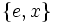# Cyclic maximal subgroup of semidihedral group:SD16

This article is about a particular subgroup in a group, up to equivalence of subgroups (i.e., an isomorphism of groups that induces the corresponding isomorphism of subgroups). The subgroup is (up to isomorphism) cyclic group:Z8 and the group is (up to isomorphism) semidihedral group:SD16 (see subgroup structure of semidihedral group:SD16).
The subgroup is a normal subgroup and the quotient group is isomorphic to cyclic group:Z2.
VIEW: Group-subgroup pairs with the same subgroup part | Group-subgroup pairs with the same group part| Group-subgroup pairs with the same quotient part | All pages on particular subgroups in groups

Here,$G$ is the semidihedral group:SD16, the semidihedral group of order sixteen (and hence, degree eight). We use here the presentation:$G := \langle a,x \mid a^8 = x^2 = e, xax = a^3 \rangle$$G$ has 16 elements:$\! \{ e,a,a^2,a^3,a^4,a^5,a^6,a^7,x,ax,a^2x,a^3x,a^4x,a^5x,a^6x,a^7x \}$

The subgroup$H$ of interest is the subgroup$\langle a \rangle$. It is cyclic of order 8 and is given by:$\! \{ e,a,a^2,a^3,a^4,a^5,a^6,a^7 \}$

## Cosets

The subgroup has index two and is hence normal (since index two implies normal). Its left cosets coincide with its right cosets, and there are two cosets:$\! H = \{ e,a,a^2,a^3,a^4,a^5,a^6,a^7 \}, G \setminus H = \{ x,ax,a^2x,a^3x,a^4x,a^5x,a^6x,a^7x \}$

## Complements

COMPLEMENTS TO NORMAL SUBGROUP: TERMS/FACTS TO CHECK AGAINST:
TERMS: permutable complements | permutably complemented subgroup | lattice-complemented subgroup | complemented normal subgroup (normal subgroup that has permutable complement, equivalently, that has lattice complement) | retract (subgroup having a normal complement)
FACTS: complement to normal subgroup is isomorphic to quotient | complements to abelian normal subgroup are automorphic | complements to normal subgroup need not be automorphic | Schur-Zassenhaus theorem (two parts: normal Hall implies permutably complemented and Hall retract implies order-conjugate)

There are four possible permutable complements to$H$ in$G$, all of them automorphic to each other:$\! \{ e, x \}, \{ e, a^2x \}, \{ e, a^4x \}, \{ e, a^6x \}$

### Properties related to complementation

Property Meaning Satisfied? Explanation Comment
complemented normal subgroup normal subgroup with permutable complement Yes see above
permutably complemented subgroup subgroup with permutable complement Yes (via complemented normal)
lattice-complemented subgroup subgroup with lattice complement Yes (via permutably complemented)
retract has a normal complement No
direct factor normal subgroup with normal complement No

### Arithmetic functions

Function Value Explanation
order of whole group 16
order of subgroup 8
index of subgroup 2
size of conjugacy class = index of normalizer 1
number of conjugacy classes in automorphism class 1

## Effect of subgroup operators

Function Value as subgroup (descriptive) Value as subgroup (link) Value as group
normalizer whole group -- semidihedral group:D16
centralizer$\langle a \rangle$ -- the subgroup itself current page cyclic group:Z8
normal core the subgroup itself current page cyclic group:Z8
normal closure the subgroup itself current page cyclic group:Z8
characteristic core the subgroup itself current page cyclic group:Z8
characteristic closure the subgroup itself current page cyclic group:Z8

## Subgroup-defining functions

Subgroup-defining function Meaning in general Why it takes this value
centralizer of derived subgroup centralizer of the derived subgroup (the commutator of the group with itself) The derived subgroup is$\langle a^2 \rangle$, which is centralized by all of$\langle a \rangle$ and by nothing outside it.

## Subgroup properties

### Invariance under automorphisms and endomorphisms

Property Meaning Satisfied? Explanation
normal subgroup invariant under inner automorphisms Yes
characteristic subgroup invariant under all automorphisms Yes On account of being the centralizer of derived subgroup, also on account of being an isomorph-free subgroup.
fully invariant subgroup invariant under all endomorphisms No not invariant under the retraction with kernel$\langle a^2, ax \rangle$ and image$\{ e, x \}$.
isomorph-free subgroup no other isomorphic subgroup Yes